# Weights and pulleys in harmonic motion

## Homework Statement

No friction. m3=5m, m2=12m, m1=3m
Prove that the system's acceleration when it's released is a=0.2g. is it the max acceleration?
Show that the equilibrium point is at x0=0.75L
Show that the kinetic energy of the system is E=mgL
Show that m2 will stop momentarily at ##x_1=\frac{15}{8}L##

## Homework Equations

Harmonic motion, the force is proportional to the displacement: F=kx
$$\ddot x=\omega^2x=0$$

## The Attempt at a Solution

The initial state: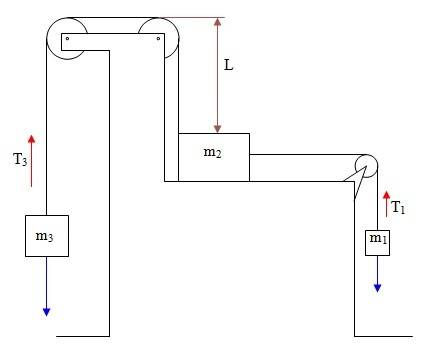$$\left\{ \begin{array}{l} m_1g-T=(m_1+m_2)a \\ T=m_2a \end{array}\right.\;\rightarrow a=\frac{3}{27}g=0.11g$$
It's wrong.
In the intermediate state: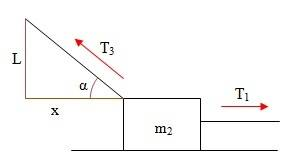m2 accelerates distance x but the rope lengthens by: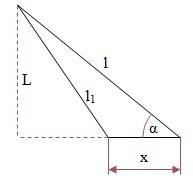The cosine rule:
$$l_1^2=x^2+\frac{L^2}{\sin^2\alpha}-2x \frac{L}{\sin\alpha}\cos\alpha$$
$$\Delta l=\frac{L}{\sin\alpha}-\sqrt{x^2-\frac{2L}{\tan\alpha}x+\frac{L^2}{\sin^2\alpha}}$$
$$\left\{ \begin{array}{l} m_1g-T_1=m_1\ddot x \\ T_1-T_3\cos\alpha=m_2\ddot\Delta l \\ T_3-m_3g=m_3\ddot\Delta l \end{array} \right.$$
It's complicated, Δl and it's derivative and i am afraid it won't get to a second order differential equation.

•arpon

ehild
Homework Helper

## Homework Statement

No friction. m3=5m, m2=12m, m1=3m
Prove that the system's acceleration when it's released is a=0.2g. is it the max acceleration?
Show that the equilibrium point is at x0=0.75L
Show that the kinetic energy of the system is E=mgL
Show that m2 will stop momentarily at ##x_1=\frac{15}{8}L##

## Homework Equations

Harmonic motion, the force is proportional to the displacement: F=kx
$$\ddot x=\omega^2x=0$$

## The Attempt at a Solution

The initial state:View attachment 88066
$$\left\{ \begin{array}{l} m_1g-T=(m_1+m_2)a \\ T=m_2a \end{array}\right.\;\rightarrow a=\frac{3}{27}g=0.11g$$
It's wrong.
The net force on m1 is m1g-T. And force = mass times acceleration.

$$\left\{ \begin{array}{l} m_1g-T=m_1a \\ T=m_2a \end{array}\right.\;\rightarrow a=\frac{3}{27}g=0.2g$$

ehild
Homework Helper
$$\left\{ \begin{array}{l} m_1g-T=m_1a \\ T=m_2a \end{array}\right.\;\rightarrow a=\frac{3}{27}g=0.2g$$
What kind of Maths do you use????

Sorry,:
$$\left\{ \begin{array}{l} m_1g-T=m_1a \\ T=m_2a \end{array}\right.\;\rightarrow a=\frac{3}{15}g=0.2g$$
And it's correct

I correct the original set of equations:
$$\left\{ \begin{array}{l} m_1g-T_1=m_1\ddot x \\ T_1-T_3\cos\alpha=m_2\ddot x \\ T_3-m_3g=m_3\ddot\Delta l \end{array} \right.$$

Last edited:
ehild
Homework Helper
I correct the original set of equations:
$$\left\{ \begin{array}{l} m_1g-T_1=m_1\ddot x \\ T_1-T_3\cos\alpha=m_2\ddot x \\ T_3-m_3g=m_3\ddot\Delta l \end{array} \right.$$
All right, so where is equilibrium?

All right, so where is equilibrium?
$$m_1g=m_3g\cdot \cos\alpha\;\rightarrow x=0.75L$$
I tried a simpler relation between x1 and x3 based on Pitagora's formula:
$$x_3=\sqrt{L^2+x^2}-L$$
$$\left\{ \begin{array}{l} m_1g-T_1=m_1\ddot x \\ T_1-T_3\cos\alpha=m_2\ddot x \\ T_3-m_3g=m_3\ddot x_3 \end{array} \right.$$
Still the derivative is complicated, maybe i don't have to derive?

ehild
Homework Helper
The second derivative of x3 is a bit complicated, but it can be done.
You can find the point where m2 momentarily stops from energy considerations.
I do not understand the question "Show that the kinetic energy of the system is E=mgL" . The kinetic energy changes during the motion. Did you copy the problem correctly?

If the system is released when x = 0 the motion is not SHM, but it can be SHM around the equilibrium point for small displacements. But I do not see any question in the problem that concerns SHM.

Show that the kinetic energy of the system is E=mgL
Indeed strange but the decrease in potential energy of m1 and 3 at the equilibrium point, at x0 is:
$$x_3=\sqrt{L^2+(0.75L)^2}-L=0.25L$$
$$E=0.75Lm_1g-0.25Lm_3g=gLm$$
You can find the point where m2 momentarily stops from energy considerations.
I guess the equilibrium point isn't in the middle of the path: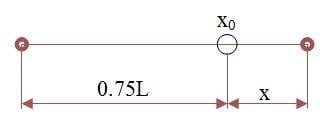The total energy of E=Lmg must be also at the right end of the path, x is the distance to the right of the equilibrium point x0:
$$Lmg=m_1gx-m_3g\left( \sqrt{L^2+(0.75x)^2}-L \right)$$
$$Lmg=m_1gx-m_3g\left( \sqrt{\frac{25}{16}+\frac{3}{2}Lx+x^2}-L \right)$$
Complicated
If the system is released when x = 0 the motion is not SHM, but it can be SHM around the equilibrium point for small displacements
How do you know that around the equilibrium point it's SHM without even seeing (or did you see) the solved equation of motion? or is every non-harmonic motion approximately SHM near the equilibrium?
I was also asked if a=0.2g, the acceleration at the start is the maximum and i think yes because x=0 is one the 2 ends of the track m2 makes and at those ends the acceleration is max

Last edited:
ehild
Homework Helper
Indeed strange but the decrease in potential energy of m1 and 3 at the equilibrium point, at x0 is:
$$x_3=\sqrt{L^2+(0.75L)^2}-L=0.25L$$
$$E=0.75Lm_1g-0.25Lm_3g=gLm$$
Then the question must have been " what is the KE at the equilibrium point". OK.
I guess the equilibrium point isn't in the middle of the path:View attachment 88357
The total energy of E=Lmg must be also at the right end of the path, x is the distance to the right of the equilibrium point x0:
$$Lmg=m_1gx-m_3g\left( \sqrt{L^2+(0.75x)^2}-L \right)$$
$$Lmg=m_1gx-m_3g\left( \sqrt{\frac{25}{16}+\frac{3}{2}Lx+x^2}-L \right)$$
Complicated

Initially you defined x as the displacement of m2 from its initial position, when the string connected to it was vertical. Write the potential energy in terms of that x. It would be much simpler. Square the equation and solve.
I considered the initial potential energy zero, and found the point where it is zero again. In this way, the equation was even simpler.

How do you know that around the equilibrium point it's SHM without even seeing (or did you see) the solved equation of motion? or is every non-harmonic motion approximately SHM near the equilibrium?

You can write the potential energy and if it has a minimum, around that minimum the Taylor series of the potential energy has a positive term proportional to the square of the displacement from the equilibrium, like in case of an SHM.

I was also asked if a=0.2g, the acceleration at the start is the maximum and i think yes because x=0 is one the 2 ends of the track m2 makes and at those ends the acceleration is max

I am not sure, and I did not do it yet. If it was SHM, the magnitude of the acceleration would be the same at both ends. But the other turning point is closer to the equilibrium point.
I suggest to find the turning point and see what forces act and what is the acceleration there.

Initially you defined x as the displacement of m2 from its initial position, when the string connected to it was vertical. Write the potential energy in terms of that x. It would be much simpler. Square the equation and solve.
$$2\cdot Lmg=m_1gx-m_3g\left( \sqrt{L^2+x^2}-L \right)\quad\rightarrow \quad 3x+3L=5\sqrt{L^2+x^2}$$
And the solution is imaginary.
I considered the initial potential energy zero, and found the point where it is zero again. In this way, the equation was even simpler.
I don't know how to do it, i think i made it in the previous equation since ##2\cdot Lmg=...##
You can write the potential energy and if it has a minimum
$$E=m_3g\left[ \sqrt{L^2+x^2}-L \right]-m_1gx$$
$$E'=\left[ \frac{m_3x}{\sqrt{L^2+x^2}}-m_1 \right]g$$
Minimum at x=0.75L
around that minimum the Taylor series of the potential energy has a positive term proportional to the square of the displacement from the equilibrium, like in case of an SHM.
The potential energy of SHM is ##E_p=\frac{1}{2}kx^2## and the Taylor series is ##f(x+h)=f(x)+hf'(x)+\frac{h^2}{2}f''(x)##
The first and second terms aren't proportional to the square of the displacement, is it enough that only the third term that is proportional and the whole potential energy will be like in SHM and the whole behavior will be like SHM?
I suggest to find the turning point and see what forces act and what is the acceleration there.
I take ##x_1=\frac{15}{8}L## as the other end, and the forces are:
$$\tan \alpha=\frac{8}{15}$$
$$F=m_3g\cos\alpha-m_1g=2.7mg$$
$$2.7mg=m_2a\quad\rightarrow \quad a=2.3g$$

ehild
Homework Helper
$$2\cdot Lmg=m_1gx-m_3g\left( \sqrt{L^2+x^2}-L \right)\quad\rightarrow \quad 3x+3L=5\sqrt{L^2+x^2}$$
And the solution is imaginary.
You do not know at what hight were the masses m1 and m3 initially. x1 and x3 are displacement from the initial positions, x1 downward and x3 upward. So what is the change of the potential energy in terms of these displacements?
When the system stops, the whole energy is potential. Initially, the system was in rest. So the potential energy at the point where the system stops is the same as it was initially. The change of potential energy is zero, not 2Lmg.
The potential energy of SHM is ##E_p=\frac{1}{2}kx^2## and the Taylor series is ##f(x+h)=f(x)+hf'(x)+\frac{h^2}{2}f''(x)##
The first and second terms aren't proportional to the square of the displacement, is it enough that only the third term that is proportional and the whole potential energy will be like in SHM and the whole behavior will be like SHM?
The equilibrium point is at the minimum of the potential energy. Take the Taylor series around that minimum. At the minimum, f ' =0. And you can choose the potential energy zero at the equilibrium point. So you have only the second-order term in the Taylor expansion.

Last edited:
The change of potential energy is zero, not 2Lmg.
$$0=m_1gx-m_3g\left( \sqrt{L^2+x^2}-L \right)\quad\rightarrow \quad x=\frac{15}{8}L$$
Initially you defined x as the displacement of m2 from its initial position, when the string connected to it was vertical. Write the potential energy in terms of that x. It would be much simpler. Square the equation and solve.
I don't get the second point:
$$Lmg=m_1gx-m_3g\left( \sqrt{L^2+x^2}-L\right)\quad\rightarrow\quad x=\frac{3}{4}L$$
And you can choose the potential energy zero at the equilibrium point. So you have only the second-order term in the Taylor expansion.
So also f(x0)=0, and i am left with:
$$E(x_0+h)=\frac{h^2}{2}f''(x_0)$$

ehild
Homework Helper
$$0=m_1gx-m_3g\left( \sqrt{L^2+x^2}-L \right)\quad\rightarrow \quad x=\frac{15}{8}L$$

That is correct.

I don't get the second point:
$$Lmg=m_1gx-m_3g\left( \sqrt{L^2+x^2}-L\right)\quad\rightarrow\quad x=\frac{3}{4}L$$
I don't get what you want with the equation above. If the kinetic energy is mgL when the potential energy is zero, then the potential energy is mgL when the kinetic energy is zero.
So also f(x0)=0, and i am left with:
$$E(x_0+h)=\frac{h^2}{2}f''(x_0)$$
I think you mean E as the potential energy, so $$E(x_0+h)=\frac{h^2}{2}E''(x_0)$$

Chestermiller
Mentor
Karol and ehild,

You guys have done an amazingly nice job of analyzing this problem. Congrats. I just have a little extra to add that I think you might find interesting.

If I combine the three force balance equations by eliminating the tensions in the two strings, I obtain the following equation:
$$m_1g-m_3g\frac{x}{\sqrt{L^2+x^2}}=(m_1+m_2)\frac{d^2x}{dt^2}+m_3\frac{x}{\sqrt{L^2+x^2}}\frac{d^2}{dt^2}\left(\sqrt{L^2+x^2}\right)$$
If I multiply this equation by dx/dt, I obtain:
$$m_1g\frac{dx}{dt}-m_3g\frac{x}{\sqrt{L^2+x^2}}\frac{dx}{dt}=(m_1+m_2)\frac{dx}{dt}\frac{d^2x}{dt^2}+m_3\left(\frac{x}{\sqrt{L^2+x^2}}\frac{dx}{dt}\right)\frac{d^2}{dt^2}\left(\sqrt{L^2+x^2}\right)$$
This equation can be reexpressed as:
$$m_1g\frac{dx}{dt}-m_3g\frac{d(\sqrt{L^2+x^2})}{dt}=\frac{(m_1+m_2)}{2}\frac{d}{dt}\left(\frac{dx}{dt}\right)^2+\frac{m_3}{2}\frac{d}{dt}\left(\frac{d(\sqrt{L^2+x^2})}{dt}\right)^2$$
Integrating this between 0 and t yields:
$$m_1gx-m_3g(\sqrt{L^2+x^2}-L)=\frac{(m_1+m_2)}{2}\left(\frac{dx}{dt}\right)^2+\frac{m_3}{2}\left(\frac{d(\sqrt{L^2+x^2})}{dt}\right)^2$$
The left hand side of this equation is minus the PE, and the right hand side is the KE.

Chet

Last edited:
•Karol
ehild
Homework Helper
Karol and ehild,

You guys have done an amazingly nice job of analyzing this problem. Congrats. I just have a little extra to add that I think you might find interesting.

If I combine the three force balance equations by eliminating the tensions in the two strings, I obtain the following equation:
$$m_1g-m_3g\frac{x}{\sqrt{L^2-x^2}}=(m_1+m_2)\frac{d^2x}{dt^2}+m_3\frac{x}{\sqrt{L^2-x^2}}\frac{d^2}{dt^2}\left(\sqrt{L^2+x^2}\right)$$
Thank you Chet!
But is not the first equation
$$m_1g-m_3g\frac{x}{\sqrt{L^2+x^2}}=(m_1+m_2)\frac{d^2x}{dt^2}+m_3\frac{x}{\sqrt{L^2+x^2}}\frac{d^2}{dt^2}\left(\sqrt{L^2+x^2}\right)$$

The problem is not completely solved yet. The question "is the initial acceleration maximum" is not answered correctly, and I think, a simple answer is expected, without deriving the formula for d2x/dt2. And the question is sloppy as the acceleration of m1 and m2 differ from that of m3.

ehild
Homework Helper
I take ##x_1=\frac{15}{8}L## as the other end, and the forces are:
$$\tan \alpha=\frac{8}{15}$$
$$F=m_3g\cos\alpha-m_1g=2.7mg$$
$$2.7mg=m_2a\quad\rightarrow \quad a=2.3g$$

I do not think it is correct. Explain, please.

Chestermiller
Mentor
Thank you Chet!
But is not the first equation
$$m_1g-m_3g\frac{x}{\sqrt{L^2+x^2}}=(m_1+m_2)\frac{d^2x}{dt^2}+m_3\frac{x}{\sqrt{L^2+x^2}}\frac{d^2}{dt^2}\left(\sqrt{L^2+x^2}\right)$$
Hi ehild,
Thanks for picking up on that typo. I've gone back and corrected it in all the equations to avoid confusing others.
The problem is not completely solved yet. The question "is the initial acceleration maximum" is not answered correctly, and I think, a simple answer is expected, without deriving the formula for d2x/dt2. And the question is sloppy as the acceleration of m1 and m2 differ from that of m3.
Here's something else weird to think about. The acceleration of masses 1 and 2 is ##d^2x/dt^2##, but the acceleration of mass 3 is
$$\frac{x}{\sqrt{L^2+x^2}}\frac{d^2x}{dt^2}+\frac{L^2}{(L^2+x^2)^{3/2}}\left(\frac{dx}{dt}\right)^2$$
So when masses 1 and 2 are not accelerating, mass 3 is accelerating (unless dx/dt = 0, which it is not). So at the "equilibrium position" x = 0.75L , mass 3 is not in equilibrium.

Chet

ehild
Homework Helper
Here's something else weird to think about. The acceleration of masses 1 and 2 is ##d^2x/dt^2##, but the acceleration of mass 3 is
$$\frac{x}{\sqrt{L^2+x^2}}\frac{d^2x}{dt^2}+\frac{L^2}{(L^2+x^2)^{3/2}}\left(\frac{dx}{dt}\right)^2$$
So when masses 1 and 2 are not accelerating, mass 3 is accelerating (unless dx/dt = 0, which it is not). So at the "equilibrium position" x = 0.75L , mass 3 is not in equilibrium.

Chet
Hi Chet,
I guess it was not the original text of the problem presented in the OP. I understood "equilibrium position" as the point (x) where the forces at all masses at rest are zero, that is all accelerations are zero. If m2 is put at that point, the system stays there.

If the system is released from the position where m2 is at the wall, it will accelerate at x=0.75L.
The mass m3 moves differently from m1 and m2. It would be interesting to see the motions in detail.

Chestermiller
Mentor
Hi Chet,
I guess it was not the original text of the problem presented in the OP. I understood "equilibrium position" as the point (x) where the forces at all masses at rest are zero, that is all accelerations are zero. If m2 is put at that point, the system stays there.
Ah...thanks.
If the system is released from the position where m2 is at the wall, it will accelerate at x=0.75L.
Actually, when m2 arrives at x = 0.75L, its acceleration will momentarily be zero, but the acceleration of m3 will not be zero at 0.75L.
The mass m3 moves differently from m1 and m2. It would be interesting to see the motions in detail.
I'm thinking of solving the problem numerically just to see. It shouldn't be too hard to do on a spreadsheet.

Chet

I do not think it is correct. Explain, please.
$$\tan \alpha=\frac{8}{15}\quad\rightarrow\quad \cos\alpha=0.88$$
$$F=m_3g\cos\alpha-m_1g=1.4mg$$
$$1.4mg=m_2a\quad\rightarrow \quad a=0.12g$$
It's not logical since the distance to the equilibrium is shorter

Chestermiller
Mentor
Hi guys,

For your consideration, I've solved the equations numerically for the velocity and the location x as a function of time. First I reduced the differential equation to dimensionless form by introducing the following dimensionless parameters:

$$X=\frac{x}{L}$$
$$T=t\sqrt{\frac{g}{L}}$$
$$V=\frac{dX}{dT}$$
$$M_1=\frac{m_1}{(m_1+m_2+m_3)}$$
$$M_3=\frac{m_3}{(m_1+m_2+m_3)}$$

Then I substituted these into the differential equations to obtain:

$$M_1-M_3\frac{X}{\sqrt{1+X^2}}\left[1+\frac{1}{[1+X^2]^{3/2}}V^2\right]=\left[1-\frac{M_3}{1+X^2}\right]\frac{dV}{dT}$$
$$\frac{dX}{dT}=V$$

The numerical solution to the equations is shown in the figures below: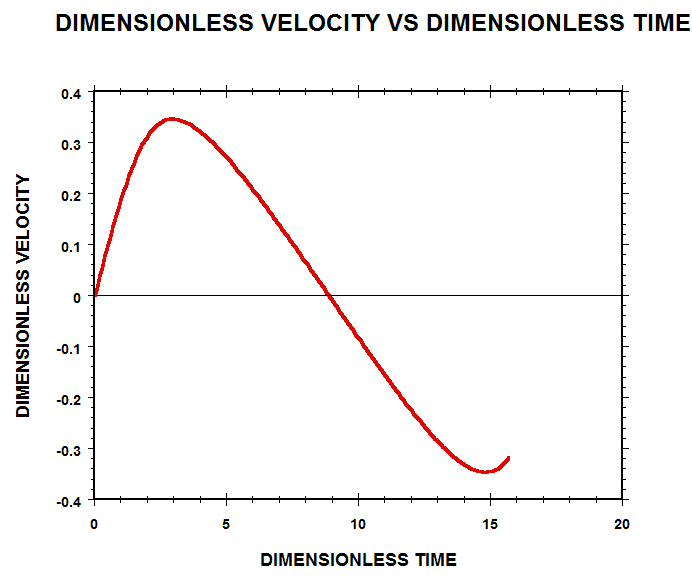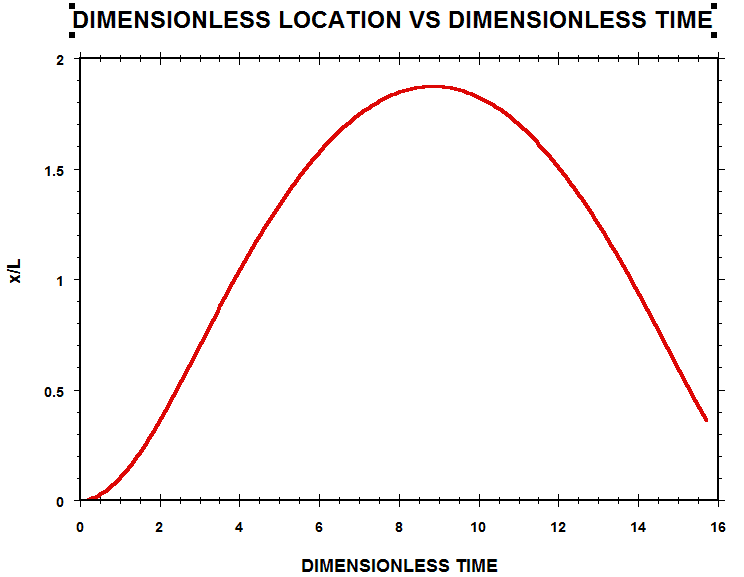Note that the maximum distance is X = 15/8=1.875

Last edited:
•ehild
ehild
Homework Helper
For your consideration, I've solved the equations numerically for the velocity and the location x as a function of time. First I reduced the differential equation to dimensionless form by introducing the following dimensionless parameters:

$$X=\frac{x}{L}$$
$$T=t\sqrt{\frac{g}{L}}$$
$$V=\frac{dX}{dT}$$
$$M_1=\frac{m_1}{(m_1+m_2+m_3)}$$
$$M_3=\frac{m_1}{(m_1+m_2+m_3)}$$

Then I substituted these into the differential equations to obtain:

$$M_1-M_3\frac{X}{\sqrt{1+X^2}}\left[1+\frac{1}{[1+X^2]^{3/2}}V^2\right]=\left[1-\frac{M_3}{1+X^2}\right]\frac{dV}{dT}$$
$$\frac{dX}{dT}=V$$

The numerical solution to the equations is shown in the figures below:

View attachment 88525 View attachment 88526
Note that the maximum distance is X = 15/8=1.875
It is amazing, Chet! It is clearly shown how asymmetric the motion is. Could you please plot the motion m1 and m3 together?

ehild
Homework Helper
$$\tan \alpha=\frac{8}{15}\quad\rightarrow\quad \cos\alpha=0.88$$
$$F=m_3g\cos\alpha-m_1g=1.4mg$$
$$1.4mg=m_2a\quad\rightarrow \quad a=0.12g$$
It's not logical since the distance to the equilibrium is shorter

The tensions acting on m2 are not m1g and m3g, as these masses are accelerating.

Also, the turning point is at distance (15/8-3/4)L from the equilibrium point, farther than the equilibrium point from the initial position.
You can see from Chet's velocity plot, that it is less steep after the equilibrium point.